# Response of AC Circuits Notes | Study Network Theory (Electric Circuits) - Electrical Engineering (EE)

## Electrical Engineering (EE): Response of AC Circuits Notes | Study Network Theory (Electric Circuits) - Electrical Engineering (EE)

The document Response of AC Circuits Notes | Study Network Theory (Electric Circuits) - Electrical Engineering (EE) is a part of the Electrical Engineering (EE) Course Network Theory (Electric Circuits).
All you need of Electrical Engineering (EE) at this link: Electrical Engineering (EE)

Finding the Response of Series RL Circuit
Consider the following series RL circuit diagram.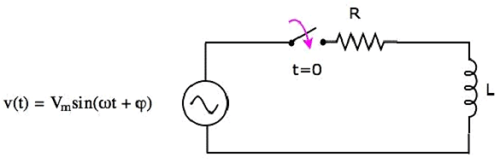In the above circuit, the switch was kept open up to t = 0 and it was closed at t = 0. So, the AC voltage source having a peak voltage of Vm volts is not connected to the series RL circuit up to this instant. Therefore, there is no initial current flows through the inductor.
The circuit diagram, when the switch is in closed position, is shown in the following figure.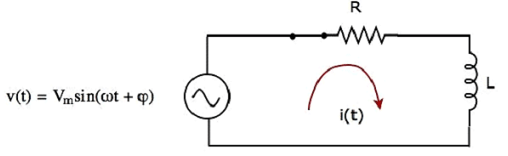Now, the current i(t) flows in the entire circuit, since the AC voltage source having a peak voltage of Vm volts is connected to the series RL circuit.
We know that the current i(t) flowing through the above circuit will have two terms, one that represents the transient part and other term represents the steady state.
Mathematically, it can be represented as
i(t) = iTr(t)+iss(t)   Equation 1
Where,

• iTr(t) is the transient response of the current flowing through the circuit.
• iss(t) is the steady state response of the current flowing through the circuit.

In the previous chapter, we got the transient response of the current flowing through the series RL circuit. It is in the form of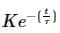Substitute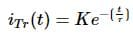in Equation 1.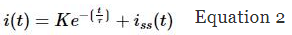If a sinusoidal signal is applied as an input to a Linear electric circuit, then it produces a steady state output, which is also a sinusoidal signal. Both the input and output sinusoidal signals will be having the same frequency, but different amplitudes and phase angles.
We can calculate the steady state response of an electric circuit, when it is excited by a sinusoidal voltage source using Laplace Transform approach.
The s-domain circuit diagram, when the switch is in closed position, is shown in the following figure.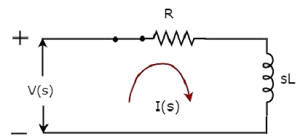In the above circuit, all the quantities and parameters are represented in s-domain. These are the Laplace transforms of time-domain quantities and parameters.
The Transfer function of the above circuit is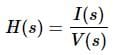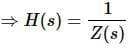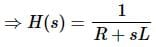Substitute s = jω in the above equation.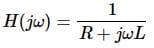Magnitude of H(jω) is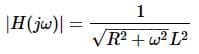Phase angle of H(jω) is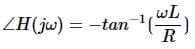We will get the steady state current iss(t) by doing the following two steps −

• Multiply the peak voltage of input sinusoidal voltage and the magnitude of H(jω).
• Add the phase angles of input sinusoidal voltage and H(jω).

The steady state current iss(t) will be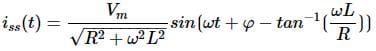Substitute the value of iss(t) in Equation 2.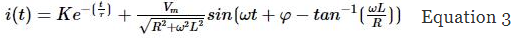We know that there is no initial current in the circuit. Hence, substitute t = 0 & i(t) = 0 in Equation 3 in order to find the value of constant, K.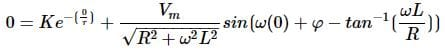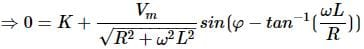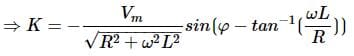Substitute the value of K in Equation 3.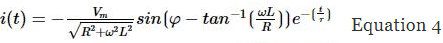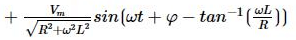Equation 4 represents the current flowing through the series RL circuit, when it is excited by a sinusoidal voltage source. It is having two terms. The first and second terms represent the transient response and steady state response of the current respectively.
We can neglect the first term of Equation 4 because its value will be very much less than one. So, the resultant current flowing through the circuit will be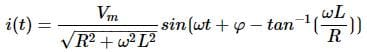It contains only the steady state term. Hence, we can find only the steady state response of AC circuits and neglect transient response of it.

The document Response of AC Circuits Notes | Study Network Theory (Electric Circuits) - Electrical Engineering (EE) is a part of the Electrical Engineering (EE) Course Network Theory (Electric Circuits).
All you need of Electrical Engineering (EE) at this link: Electrical Engineering (EE)Use Code STAYHOME200 and get INR 200 additional OFF

## Network Theory (Electric Circuits)

23 videos|21 docs|27 tests

Track your progress, build streaks, highlight & save important lessons and more!

,

,

,

,

,

,

,

,

,

,

,

,

,

,

,

,

,

,

,

,

,

;